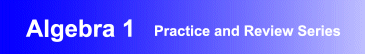Award-Winning

Algebra 1 Academic Instructional Review and Practice Software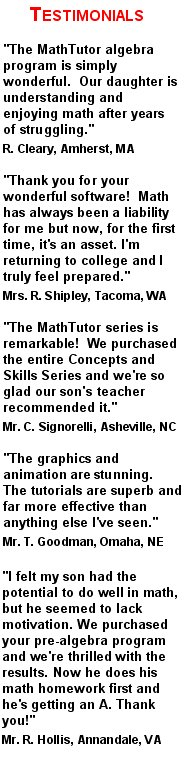DESCRIPTION
ALGEBRA 1 is the ideal

It is

algebra educational software

at its finest.
Parents wanting homeschool algebra 1 drill and practice instructional software will find the program to be perfectly suited to their needs.
Schools seeking

curriculum-based algebra 1 practice and review academic instructional software

will find the program to be ideal.
And individuals wanting an interactive algebra 1 practice and drill computer package designed for self study will find the program to be exactly what they were looking for.
ALGEBRA 1 has been acclaimed for its educational excellence as well as its flexibility. It can be used for self-study and to supplement classroom instruction in high school and middle school math classes.
Designed by math educators and in accordance with math curriculum standards, the program provides interactive algebra 1 drill and practice exercises suitable for students at all levels of ability.
Examples and algebra 1 problem solving exercises progress gradually from the simpler to the more challenging, allowing students to develop outstanding algebra 1 problem solving skills.
• Award-winning, curriculum-based algebra 1 drill and practice software developed by math educators
• Provides dynamic, interactive algebra 1 problem solving exercises that are ideal for self-study, and as a supplement to classroom instruction
• Demonstrated success in boosting students' math skills and comprehension, and proficiency in problem solving
• Network versions contain student record-keeping, grading, and performance analysis features
AVAILABLE FOR WINDOWS
• Windows XP/Vista
• Windows 7/8
• Windows 10
PRICING Single User Version: \$49.95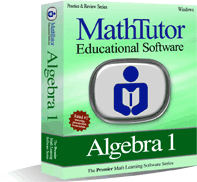TOPICS COVERED
Review of Arithmetic
• Basic Rules of Arithmetic
• Add., Subtr., Mult., Division of Fractions
• Comparison of Fractions
• Interpretation of Fractions
• Arithmetic Comparisons
• Scientific Notation
Simple Algebraic Operations
• Addition and Subtr. of Polynomials
• Multiplication of Polynomials
• Elementary Factoring I
• Elementary Factoring II
• Addition and Subtr. of Rational Expressions
• Solving Simple Algebraic Equations I
• Solving Simple Algebraic Equations II
• Units of Measure
• D = RT
• Elementary Word Probs I: Linear Eqns
• Elementary Word Probs II: Quad. Eqns
Operations

• Intermediate Factoring
• Finding the Roots of a Quadratic Equation
• Combining Algebraic Expressions I
• Combining Algebraic Expressions II
• Exponent Rules
• Pythagorean Theorem
• Intermediate Word Problems I
• Intermediate Word Problems II
Functions and Miscellaneous Topics
• Simple Word Problems
• Coordinate Geometry
• Lines in the Plane
• Equation of a Straight Line
• Solving an Inequality
• Absolute Value Function
• Determining a Function
• Direct and Inverse Proportions
• Word Problems: Absolute Value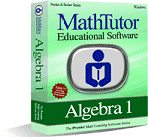Order NowTOPICS COVERED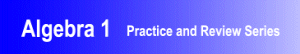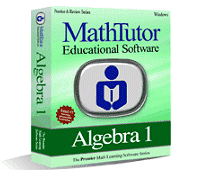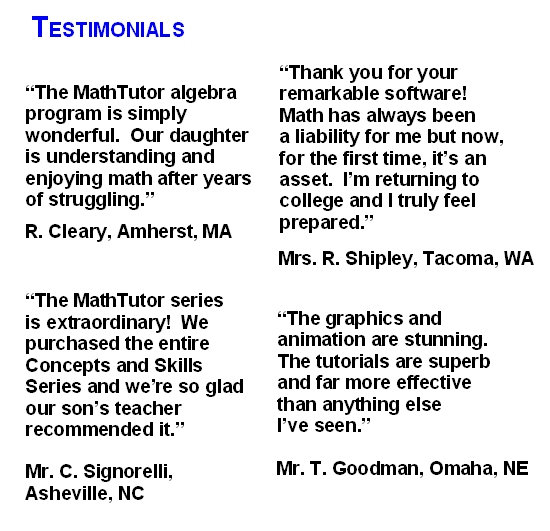TOPICS COVERED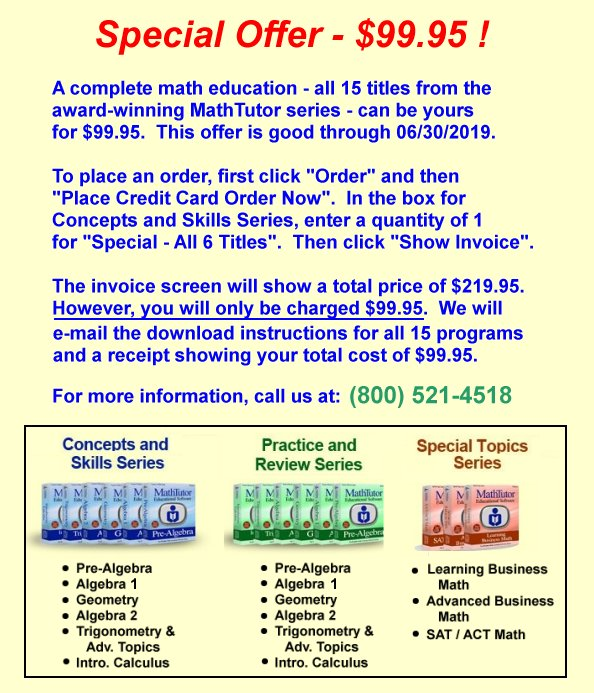×